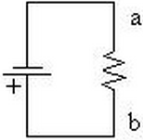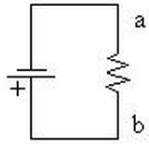# Problem: (a) In the attached figure, the (conventional) current through the resistor will go A. from a to b.B. from b to a.(b) Electrons moving through the circuit in the attached figure will go through the resistorA. go from b to a B. go from a to b

###### FREE Expert Solution

(a) Conventional currents behave as if positive charge carriers cause current to flow. Therefore, conventional currents flow from the positive terminal to the negative terminal of the cell.

97% (447 ratings)###### Problem Details

(a) In the attached figure, the (conventional) current through the resistor will goA. from a to b.

B. from b to a.

(b) Electrons moving through the circuit in the attached figure will go through the resistorA. go from b to a

B. go from a to b